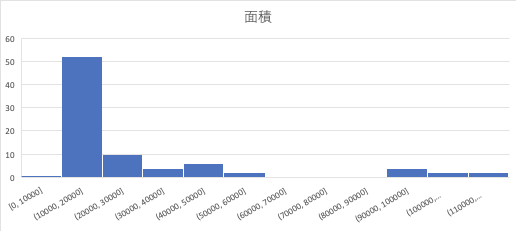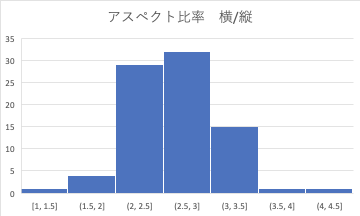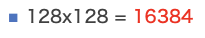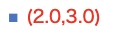# Bbox optimization on custom datasets

When inferring using self-data in maskrcnn etc., optimization can be done if there is only one category.

For example, if the length and width are predetermined, or if the size is roughly known before inference, when generating the anchor box Creating something close to that shape will lead to improvements in calculation cost and accuracy.

When an anchor box is created in the FPN layer

The initial size is (0.5,1.0,2.0) The aspect ratio is (32, 64, 128, 256, 512)

In order to estimate which size is good, the area and aspect ratio are converted into a histogram using the information of the polygon of the annotated mask.

``````import IPython
import os
import json
import random
import numpy as np
import requests
from io import BytesIO
from math import trunc
from PIL import Image as PILImage
from PIL import ImageDraw as PILImageDraw
import base64
import pandas as pd
import numpy as np

class CocoDataset():
def __init__(self, annotation_path, image_dir):
self.annotation_path = annotation_path
self.image_dir = image_dir
self.colors = colors = ['blue', 'purple', 'red', 'green', 'orange', 'salmon', 'pink', 'gold',
'orchid', 'slateblue', 'limegreen', 'seagreen', 'darkgreen', 'olive',
'teal', 'aquamarine', 'steelblue', 'powderblue', 'dodgerblue', 'navy',
'magenta', 'sienna', 'maroon']

json_file = open(self.annotation_path)

json_file.close()

self.process_categories()
self.process_images()
self.process_segmentations()

def display_info(self):
print('Dataset Info:')
print('=============')
for key, item in self.info.items():
print('  {}: {}'.format(key, item))

requirements = [['description', str],
['url', str],
['version', str],
['year', int],
['contributor', str],
['date_created', str]]
for req, req_type in requirements:
if req not in self.info:
print('ERROR: {} is missing'.format(req))
elif type(self.info[req]) != req_type:
print('ERROR: {} should be type {}'.format(req, str(req_type)))
print('')

print('=========')

requirements = [['id', int],
['url', str],
['name', str]]
print('  {}: {}'.format(key, item))
for req, req_type in requirements:
print('ERROR: {} is missing'.format(req))
print('ERROR: {} should be type {}'.format(req, str(req_type)))
print('')
print('')

def display_categories(self):
print('Categories:')
print('=========')
for sc_key, sc_val in self.super_categories.items():
print('  super_category: {}'.format(sc_key))
for cat_id in sc_val:
print('    id {}: {}'.format(cat_id, self.categories[cat_id]['name']))
print('')

def print_segmentation(self):
self.segmentations = {}
data = []
for segmentation in self.coco['annotations']:
area = segmentation['area']
print(area)
bbox = segmentation['bbox']
aspect = bbox/bbox
print(bbox)
print(aspect)
data.append([area,aspect])
df = pd.DataFrame(data)
df.to_csv('out.csv')
print(df)

annotation_path = "Annotated coco json file path"
image_dir = 'The path of the folder where the images are stored'
coco_dataset = CocoDataset(annotation_path, image_dir)
coco_dataset.print_segmentation()
``````

If you do this, you will get a csv with the area and aspect ratio as shown below.After that, I will graph it with Excel.In this case, you can see that the area is often around 10000.So this time I know that the 128 anchor size is important.

You can see that it would be nice if we could make 256 or 512 that is larger than that size. On the contrary, it turns out that the size of 64 is almost nonexistent, so it can be deleted.

If you think about the aspect ratio in the same way, you can see that there are very few places where the height: width is 0.5 this time.You can see that you should set it around.

## Summary

With the above method, you can find the optimum parameter for the anchor box when there are few categories. I hope it can be optimized.

Recommended Posts